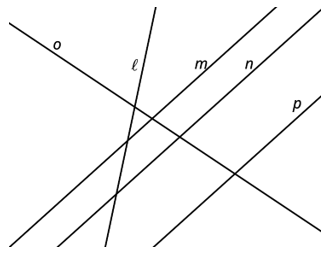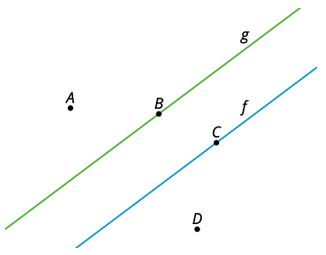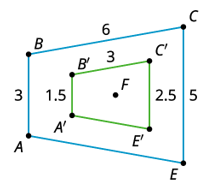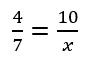# Unit 3 Lesson 4 Practice

Angle ABC is taken by a dilation with center P and scale factor 3 to angle A'B'C'. The measure of angle ABC is 21 degrees. What is the measure of angle A'B'C'?

Select all lines that could be the image of line m by a dilation.Select all that apply
• A
• B
• C
• D
• E
Check my answer (3)

Dilate line f with a scale factor of 2. The image is line g. Which labeled point could be the center of this dilation?Select all that apply
• A
• B
• C
• D
• E
Check my answer (3)

Quadrilateral A'B'C'E' is the image of quadrilateral ABCE after a dilation centered at F. What is the scale factor of this dilation?A polygon has a perimeter of 18 units. It is dilated with a scale factor of 3/2. What is the perimeter of its image?

Select all that apply
• A
• B
• C
• D
Check my answer (3)

Solve the equation.